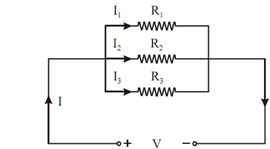## Current Division Rule Assignment Help

Assignment Help: >> Voltage and Current Division in Resistive Circuits - Current Division Rule

Current Division Rule:

Current,       I1 =V/R1But,

V = I . Req

∴          I1 = I . (Req /R1)  = I. (R2 R3 /(R1 R2  + R2 R3 + R3 R1)

Similarly

I2   = I .             R1 R3  / (R1 R2 + R 2 R3 + R3  R1)

And, I3  = I .      R1 R2    /(R1 R2 + R 2 R3 + R3  R1)